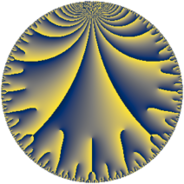# Properties

 Label 95.11.cLevel $95$ Weight $11$ Character orbit 95.c Rep. character $\chi_{95}(56,\cdot)$ Character field $\Q$ Dimension $68$ Newform subspaces $1$ Sturm bound $110$ Trace bound $0$

# Related objects

## Defining parameters

 Level: $$N$$ $$=$$ $$95 = 5 \cdot 19$$ Weight: $$k$$ $$=$$ $$11$$ Character orbit: $$[\chi]$$ $$=$$ 95.c (of order $$2$$ and degree $$1$$) Character conductor: $$\operatorname{cond}(\chi)$$ $$=$$ $$19$$ Character field: $$\Q$$ Newform subspaces: $$1$$ Sturm bound: $$110$$ Trace bound: $$0$$

## Dimensions

The following table gives the dimensions of various subspaces of $$M_{11}(95, [\chi])$$.

Total New Old
Modular forms 102 68 34
Cusp forms 98 68 30
Eisenstein series 4 0 4

## Trace form

 $$68 q - 35644 q^{4} - 2044 q^{6} + 76620 q^{7} - 1353552 q^{9} + O(q^{10})$$ $$68 q - 35644 q^{4} - 2044 q^{6} + 76620 q^{7} - 1353552 q^{9} - 418144 q^{11} + 21763300 q^{16} - 10023236 q^{17} + 518264 q^{19} + 4962204 q^{23} + 2604244 q^{24} + 132812500 q^{25} + 32178588 q^{26} - 109025284 q^{28} + 39875000 q^{30} + 39875000 q^{35} + 420547696 q^{36} - 504912596 q^{38} + 51693988 q^{39} + 343589380 q^{42} + 72617360 q^{43} - 846875584 q^{44} + 148625000 q^{45} + 426767656 q^{47} + 3802641528 q^{49} + 594603476 q^{54} - 361360620 q^{57} - 1963881788 q^{58} + 7470512872 q^{61} - 2514646024 q^{62} - 6611740944 q^{63} - 13094608884 q^{64} + 9558616040 q^{66} + 17177918780 q^{68} - 2447766556 q^{73} - 1842337832 q^{74} - 5604056776 q^{76} - 320485400 q^{77} - 821875000 q^{80} + 5162401956 q^{81} - 17137563304 q^{82} - 14922506320 q^{83} - 4799500000 q^{85} + 5085654996 q^{87} - 27413957236 q^{92} - 11067885256 q^{93} + 1613500000 q^{95} - 67563054044 q^{96} + 76070139768 q^{99} + O(q^{100})$$

## Decomposition of $$S_{11}^{\mathrm{new}}(95, [\chi])$$ into newform subspaces

Label Dim $A$ Field CM Traces $q$-expansion
$a_{2}$ $a_{3}$ $a_{5}$ $a_{7}$
95.11.c.a $68$ $60.359$ None $$0$$ $$0$$ $$0$$ $$76620$$

## Decomposition of $$S_{11}^{\mathrm{old}}(95, [\chi])$$ into lower level spaces

$$S_{11}^{\mathrm{old}}(95, [\chi]) \cong$$ $$S_{11}^{\mathrm{new}}(19, [\chi])$$$$^{\oplus 2}$$